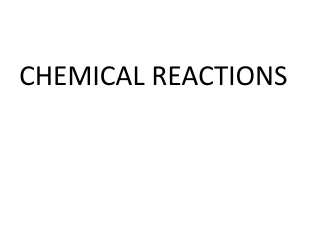DownloadDownload PresentationCHEMICAL REACTIONS

# CHEMICAL REACTIONS

Download Presentation## CHEMICAL REACTIONS

- - - - - - - - - - - - - - - - - - - - - - - - - - - E N D - - - - - - - - - - - - - - - - - - - - - - - - - - -
##### Presentation Transcript

1. CHEMICAL REACTIONS

2. Processes in which the atoms of one or more substances are rearranged to form different chemical compounds

3. Chemical reactions involve the breaking of chemical bonds in the reactants and the formation of new bonds in the products.

4. Signs of chemical reactions • Temperature changes • Exothermic • endothermic • Gas produced • Solid is formed-precipitate • Spontaneous Color change

5. Reactants are • the starting substances of a chemical reaction

6. Products are • the substances that are formed during the reaction

7. Reactants Products

8. A chemical equation is a representation of a chemical reaction in which reactants and products are expressed as formulas

9. Types of Equations • Word • Skeleton equation • Balanced equation

10. Sodium reacts with chlorine gas to make sodium chlorideNa + Cl2  NaCl2 Na + Cl2 2 NaCl

11. Symbols in Equations •  • + • (s) • (l) • Yields, makes produces • Reversible reaction • And, reacts with,combines with • Solid • Liquid-only water and bromine

12. Symbols in Equations (cont) • (aq) • (g) • Arrow up • Arrow down • Aqueous(dissolved in water) • Gas • Gas as product • Precipitate formed

13. Symbols in Equations (cont) • Heat is supplied to reaction • A catalyst is needed

14. Catalyst-a substance that speeds up the rate of a reaction but is not used up in a reactionnot a reactant or product, so written above or below arrow

15. 2Na(s) + Cl2(g)  2NaCl(s)

16. The Law of Conservation of Mass • Mass is neither created or destroyed in a chemical reaction • Mass of reactants = mass of products

17. In order to show that mass is conserved during a reaction, a chemical equation must be balanced by adding coefficients.

18. A coefficient is a • Small whole number in front of a formula

19. Steps to balancing • Write correct formulas and make skeleton equation • Count the number of atoms of each element on both reactant and product side • Balance by using coefficients NOT subscripts • MNIOH • Make sure all coefficients are in the lowest possible ratio

20. Na + Cl2 NaCl

21. Practice sheet